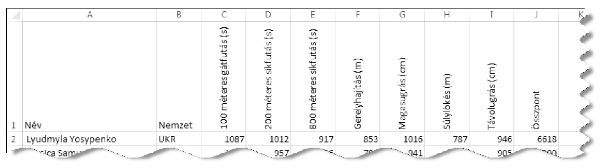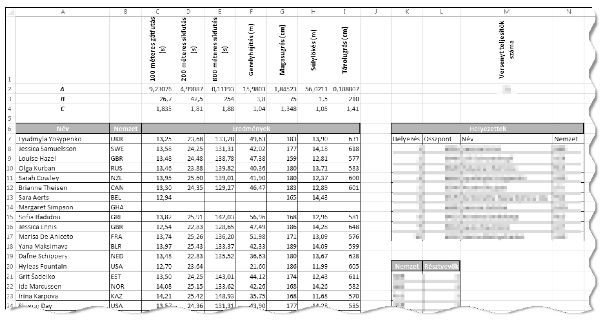Mathematical and Physical Journal
for High Schools
Issued by the MATFUND Foundation
 Already signed up? New to KöMaL?

#Problem I. 389. (December 2015)

I. 389. Women's heptathlon is a combined athletics event. This has been the topic of our database management problem I. 338. Now you should evaluate the results of a similar event by using a spreadsheet application.

The seven events of a heptathlon are the following: 100 meters hurdles, 200 meters and 800 meters track running, high jump, long jump, shot put, and javelin throw. Points are awarded based on a specific formula and on an international standard performance table according to the following.

$\displaystyle \text{Points obtained}=\big[A\cdot {|X-B|}^C\big],$

where $\displaystyle X$ is the athlete's performance, $\displaystyle [~]$ denotes the integer part, and $\displaystyle |~|$ is the absolute value. The constants $\displaystyle A$, $\displaystyle B$ and $\displaystyle C$ are determined by the International Association of Athletics Federations, and depend on the type of the event:

 Event $\displaystyle A$ $\displaystyle B$ $\displaystyle C$ 200 m running 4.990870 42.5 1.810 800 m running 0.111930 254.0 1.880 100 m hurdles 9.230760 26.7 1.835 High jump 1.845230 75.0 1.348 Long jump 0.188807 210.0 1.410 Shot put 56.02110 1.5 1.050 Javelin throw 15.98030 3.8 1.040

The tabulator-separated and UTF-8-encoded file hetforras.txt contains data corresponding to the heptathlon events during the 2012 Summer Olympics, and the constants from the previous table.

1. Import the text file hetforras.txt into the spreadsheet application beginning with cell A1. (The range A1:I4 contains the transpose of the above table.) The name of the sheet should be eredmények (=results). Your work should be saved as hetproba (=hepathlon) in the default file format of the spreadsheet application.

2. Create a new sheet pontszámok (=scores), and create a header in the first row according to the sample.3. In the range A2:B40 of this sheet-referencing cells A7:B45 of the sheet eredmények-display the name (=Név) and nationality (=Nemzet) of the 39 athletes.

4. In cells C2:I40, according to the method described above and by using a single formula and its copied version, you should determine the points for each athlete and for each event. If the corresponding eredmények cell is empty, then the referencing pontszámok cell should also be empty.

5. In cells J2:J40, for each athlete, you should give the sum of the points obtained for the events-provided that they have results in all seven categories; otherwise, a message Nincs'' (=none) should appear.

The following tasks should be solved by using the sheet eredmények.

6. In cell M2 and by using a function, you should determine the number of athletes who completed the heptathlon (=Versenyt teljesítők száma), that is, having points in all seven categories.

7. In cells K8:N17 and by using a formula, you should list the number of points (=Összpont), the name of the athlete (=Név), and the abbreviation of their nationality (=Nemzet) for the first 10 athletes (ranked according to their points (=Helyezés), which can be assumed to be different for different athletes).

8. In cells C50:I50, determine the names of the winners for each event. If there is a tie in a particular category, it is sufficient to give only one athlete name. 9. It is possible that more athletes participate in the heptathlon (=Résztvevők) from the same nation (=Nemzet). Under the cells K20:L20, you should list the number of athletes (sorted in decreasing order) for each nation. In column K in your solution, you can collect and use the 3-letter abbreviations of the nations-it is not required to use any formula. 10. Cells of the sheet eredmények should be formatted according to the sample sheet.The spreadsheet (hetproba.xlsx, hetproba.odt) and a short documentation (also with the name and version number of the spreadsheet application) should be submitted in a compressed file i389.zip.